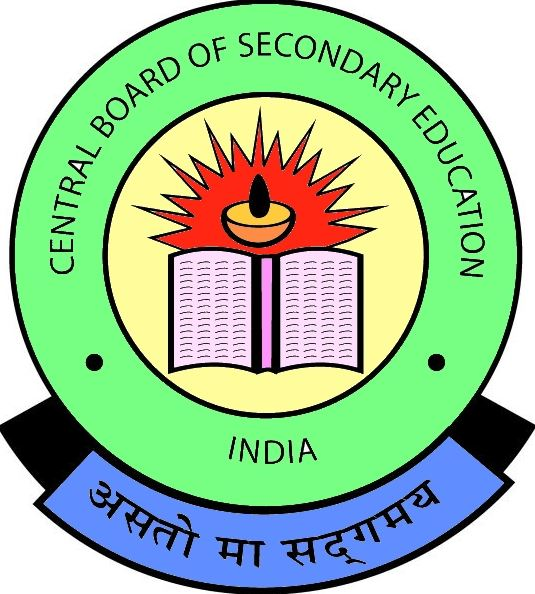1. /
2. CBSE
3. /
4. Class 12
5. /
6. CBSE Class 12 Chemistry...

# CBSE Class 12 Chemistry Sample Papers 2015

CBSE Class 12 Chemistry Sample Papers 2015 as per the latest revised marking scheme and question paper pattern issued by CBSE for class 12 chemistry is now available for free download in PDF file format at myCBSEguide.com.

##http://mycbseguide.com

## CBSE Class 12 Chemistry Sample Papers 2015

These sample question papers are prepared by our team of expert teachers. Class-12 Chemistry syllabus has some changes in marking scheme and question paper design. The new question paper for 2015 onwards, there will be 26 questions. Last year there was 30 questions in all.Test Generator

Create question paper PDF and online tests with your own name & logo in minutes.myCBSEguide

Question Bank, Mock Tests, Exam Papers, NCERT Solutions, Sample Papers, Notes

### 2 thoughts on “CBSE Class 12 Chemistry Sample Papers 2015”

1. is any body tell me about rault’s law

2. If you have a solution of volatile liquid,then the vapour pressure exerted by each gas is directly proportional to their mole fraction.
By henry’s law
p1=p1(knot)x1
p2=p2(knot)x2
By rault’s law,P(total)=p1+p2
=p1(knot)x1+p2(knot)x2
=p1(knot)x1+p2(knot)(1-x1) [since x1+x2=1]
then just simplify it a bit more to obtain the formula.